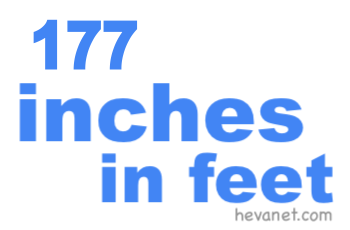177 inches in feetHere we will show you how to get 177 inches in feet (177 in to ft). We will show you how to convert 177 inches in feet as a decimal, 177 inches in feet and inches as a decimal, 177 inches in feet as a fraction, and finally, 177 inches in feet and inches as a fraction.

177 inches in feet as a decimal
We start with the easiest conversion. There are twelve inches per foot. Thus, to convert 177 inches to feet as a decimal, you can simply divide 177 by 12 like this:

177 ÷ 12 = 14.75
177 inches = 14.75 feet

177 inches in feet and inches as a decimal
177 inches makes 14 full feet per our calculation above. We subtract the full feet from 177 to get the remaining inches. Below is the math and the answer.

177 - (14 × 12) = 9
177 inches = 14 feet and 9 inches

177 inches in feet as a fraction
You already have the whole number of feet from the answer above. To get the fractional number in feet, you put the decimal inches number from the answer above over 12 and then simplify:

9/12 = 90/120 = 3/4
177 inches = 14 3/4 feet

177 inches in feet and inches as a fraction
Again, you already have the whole number of feet from the answer above. To get the fractional inches, you put the decimal inches number from the answer above over 1 and then simplify:

9/1 = 90/10 = 9
177 inches = 14 feet and 9 inches

Inches in Feet
Enter another length of inches below to see what it is in feet.

177.1 inches in feet
Go here for the next length in inches on our list that we have converted to feet for you.